# Calculate Areas: Triangles

In this worksheet, students must calculate the areas of the given triangles.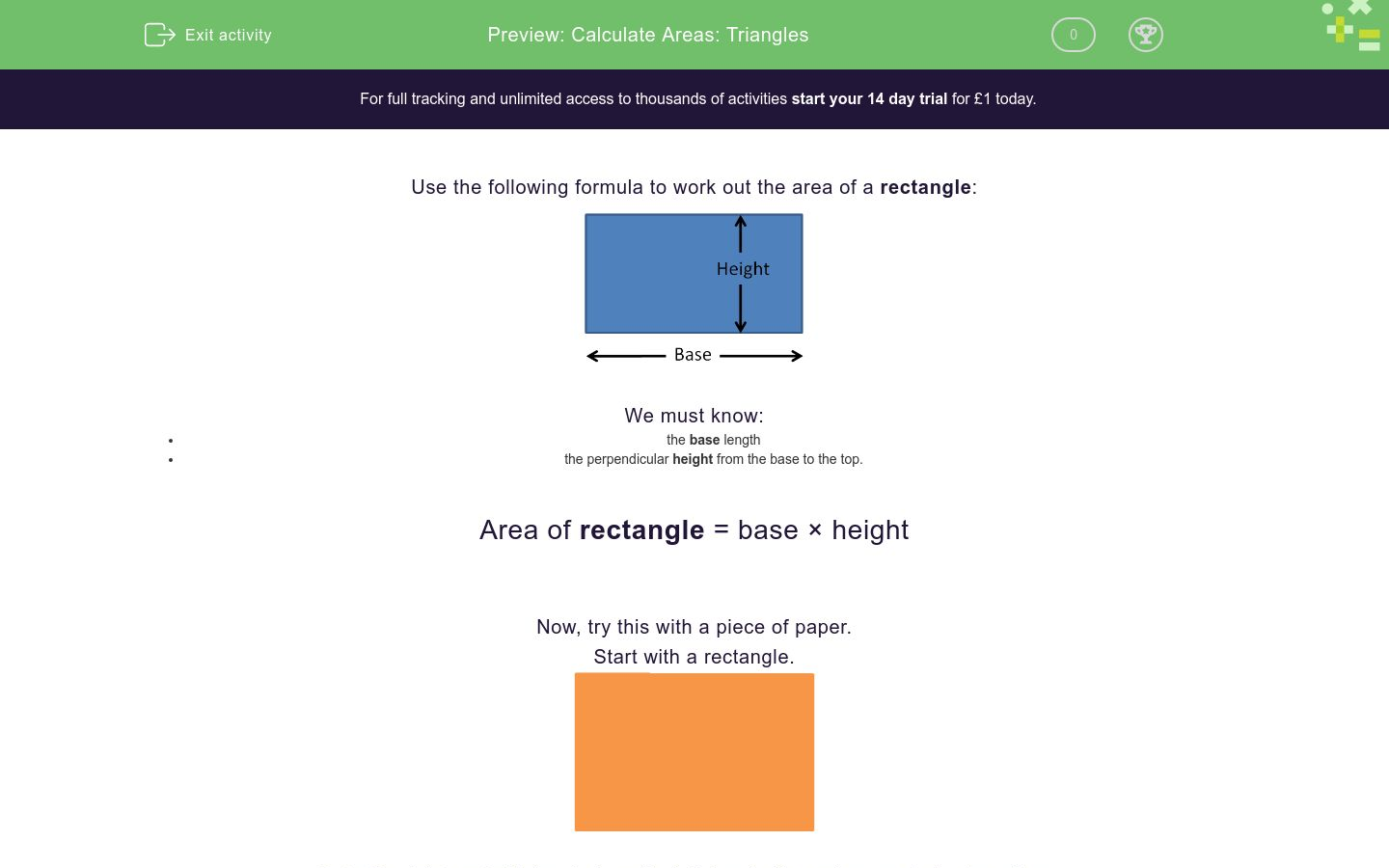Key stage:  KS 2

Curriculum topic:   Measurement

Curriculum subtopic:   Calculate Areas

Difficulty level:### QUESTION 1 of 10

Use the following formula to work out the area of a rectangle: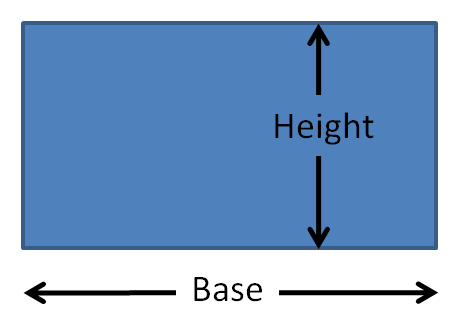We must know:

• the base length
• the perpendicular height from the base to the top.

Area of rectangle = base × height

Now, try this with a piece of paper.Cut off a right-angled triangle from the left-hand side and separate the two pieces.

The area of the two pieces together will be the same as the original rectangle.Cut off a right-angled triangle from the right-hand side to form a triangle in the middle, and separate the three pieces.

The area of the three pieces will still be the same as the original rectangle.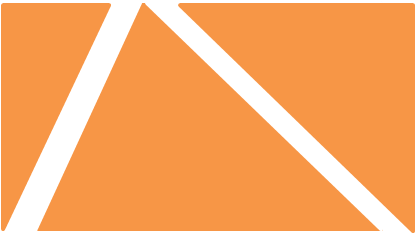Now look carefully at the pieces marked A and B below.

Notice that the areas match.

A matches A and B matches B.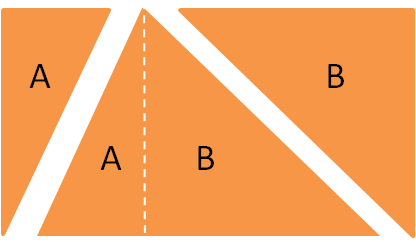So the area of the triangle in the middle is half the area of the rectangle.

Area of TrianglesWe must know:

• the base length
• the perpendicular height from the base to the apex. (NOT the slant heights)

Area of triangle = ½ base × height

or

Area of triangle = base × height ÷ 2

Example

Calculate the area of this triangle in cm2.This is a triangle.

We must know:

• the base length
• the perpendicular height from the base to the top. (NOT the slant height)

If we turn our heads to the left, the base length is 12 cm and the perpendicular height is 6 cm.

We do not need to know the slant height of 10 cm to use the formula.

Now we use the area formula:

Area of triangle = ½ base × height

Area = ½ × 12 × 6 = 6 × 6  =  36 cm2

Or...

Area of triangle = base × height ÷ 2

Area = 12 × 6  ÷ 2 = 72 ÷ 2 =  36 cm2

Select the correct formula to calculate the area of a triangle.

area = base × perpendicular height

area = ½ base × perpendicular height

area = base × slant height

Calculate the area of this triangle in cm2.

(Just write the number.)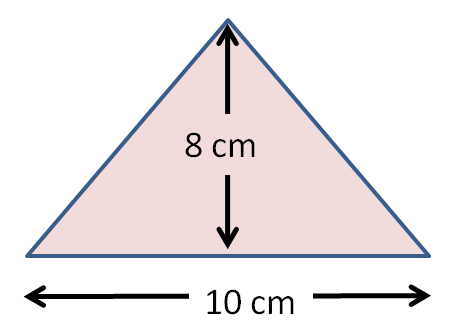Calculate the area of this triangle in cm2.

(Just write the number.)Calculate the area of this triangle in cm2.

(Just write the number.)Calculate the area of this right-angled triangle in cm2.

(Just write the number.)Calculate the area of this triangle in cm2.

(Just write the number.)Which of these two triangles has the larger area?

The base lengths and the perpendicular heights are shown.A

B

cannot tell

Which of these two triangles has the larger area?

The base lengths and the perpendicular heights are shown.A

B

cannot tell

Which of these two triangles has the larger area?A

B

cannot tell

Is this statement true or false?

"These triangles have the same area."true

false

cannot tell

• Question 1

Select the correct formula to calculate the area of a triangle.

area = ½ base × perpendicular height
EDDIE SAYS
This is the same as base × height ÷ 2.
• Question 2

Calculate the area of this triangle in cm2.

(Just write the number.)40
EDDIE SAYS
Base length = 10 cm
Perpendicular height = 8 cm
Area = 10 × 8 ÷ 2 = 80 ÷ 2 = 40 cm2
• Question 3

Calculate the area of this triangle in cm2.

(Just write the number.)42
EDDIE SAYS
Base length = 12 cm
Perpendicular height = 7 cm
Area = ½ × 12 × 7 = 6 × 7 = 42 cm2
• Question 4

Calculate the area of this triangle in cm2.

(Just write the number.)35
EDDIE SAYS
Base length = 14 cm
Perpendicular height = 5 cm
Area = ½ × 14 × 5 = 7 × 5 = 35 cm2
• Question 5

Calculate the area of this right-angled triangle in cm2.

(Just write the number.)60
EDDIE SAYS
Base length = 15 cm
Perpendicular height = 8 cm
Area = 15 × 8 ÷ 2 = 15 × 4 = 60 cm2
• Question 6

Calculate the area of this triangle in cm2.

(Just write the number.)54
EDDIE SAYS
Base length = 12 cm
Perpendicular height = 9 cm
Area = 12 × 9 ÷ 2 = 108 ÷ 2 = 54 cm2
• Question 7

Which of these two triangles has the larger area?

The base lengths and the perpendicular heights are shown.A
EDDIE SAYS
Same base lengths, but the height of A is more.
So the area of A will be bigger.
• Question 8

Which of these two triangles has the larger area?

The base lengths and the perpendicular heights are shown.B
EDDIE SAYS
Area of A = 12 × 10 ÷ 2 = 60 cm2
Area of B = 16 × 8 ÷ 2 = 64 cm2
• Question 9

Which of these two triangles has the larger area?cannot tell
EDDIE SAYS
Area of B = 5 × 7 = 35 cm2
We cannot work out the area of A because the perpendicular height is not shown.
• Question 10

Is this statement true or false?

"These triangles have the same area."true
EDDIE SAYS
Area of top triangle = 10 × 8 ÷ 2 = 40 cm2
Area of bottom triangle = 16 × 5 ÷ 2 = 40 cm2
---- OR ----